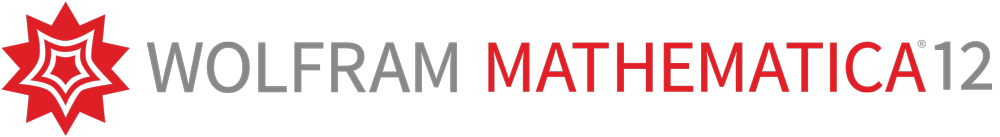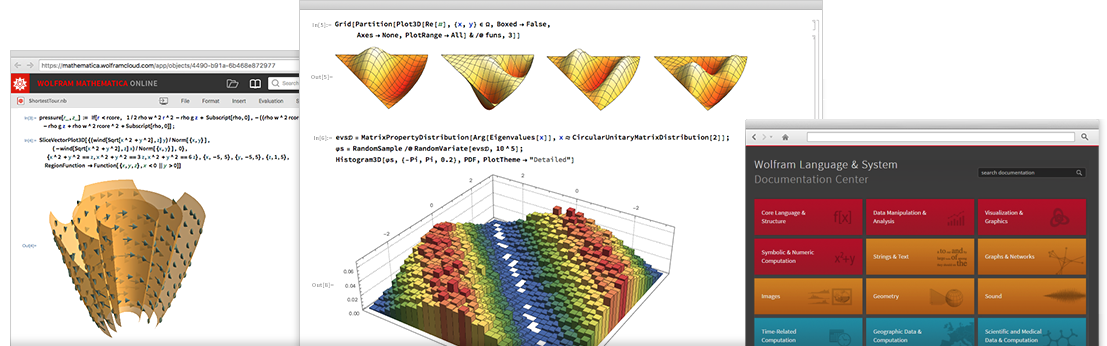# Wolfram Mathematica# Wolfram Mathematica

## Compute • Develop • Deploy

Mathematica is the integrated system for the complete development, computation, simulation, analysis, and documentation of technical problems in a unified, platform-independent working environment using Wolfram Language. With the revolutionary "one-click" runtime creation for passing on results with the Wolfram CDF Player's technology – an unequalled simple workflow – Mathematica is the ideal technical development environment using automated runtime creation. Mathematica integrates parallelised technologies to use multicore PCs or GPUs to full capacity and enables the generation of C code.

Mathematica provides the user with an almost infinite variety of mathematical functions to solve tasks from the fields of engineering, finance and insurance mathematics, mathematics, statistics, physics, "chemical computing" and, of course, all natural sciences. Additionally, it is possible to extend Mathematica's "knowledge" at will.

The user interacts with Mathematica via its intuitive user interface, the Notebook interface, that provides a clear and structured presentation of texts, formulas, models and simulations and even dynamic objects in an interface.The integrated machine learning module allows the automated growth of neural nets in unprecedented amorphous learning structures. Due to its multicore support, it is even possible to compute very large networks, since Mathematica has no memory limits.

The extensive algorithms for 2D and 3D image analysis, including 3D volume rendering, allow for in-depth insights into complex images and geometries. Using a 3D printer, users can print 3D elements true to the model with the 3D printing feature.

By means of filters and special effects users can perform a more detailed analysis of audio and speech data. They can also synthetize any data, overlayed by filters and special effects.

For the field of linguistics and the associated speech analysis, the developers integrated a pool of functions to enable even the most complex text/string research. Some virtual assistants use these functions for live analysis and presentation of results. In computer linguistics, users can automate the visualization of, e.g., word clouds, grammar trees and, also, constituent trees.

Geodata in computations, analyses and visualizations is easy to handle for Mathematica, because it is nothing else than computable knowledge. Therefore, Mathematica is the ideal tool for the wide field of geomapping, which is connecting data with digital maps.

Speziell für den Bereich der neuen Technologien Blockchain und auch Kryptowährungen haben die Entwickler eigene Algorithmen entwickelt, die es erlauben, mit diesen Dingen umzugehen sowie die umfangreichen Informationen zu verarbeiten und weiterzubearbeiten.

Especially for new technologies as blockchain and, also, cryptocurrencies, the developers came up with their own algorithms, enabling to work in these fields and to further process the extensive information.

Another expert area in Mathematica is the extended knowledge of anatomy of the human body and many animal species as 3D models and data (position, connection, mass, ...).

Mathematica is the system for everything containing computable knowledge and allows for passing on this knowledge for free via CDF technology.

### Core Technologies

• Wolfram Language
The knowledge-based symbolic language that grew out of Mathematica, and now powers the Mathematica system.
• Functions & Algorithms
Mathematica offers an almost infinte amount of algorithms and functions covering the most different areas.
• Wolfram Knowledgebase – integriertes Wissen
The broad, continuously updated knowledgebase that powers Wolfram|Alpha and supplies computable real-world data for use in Wolfram products.
• Compute As You Think Technologie
Plain English can be used as input, based on Wolfram|Alpha.
• Wolfram Predictive Interface
The Wolfram Predictive Interface offers the user predictive suggestions and is based on the analysis of millions of queries to Wolfram|Alpha.
• Notebook Concept
The flexible document-based interface that lets you mix executable code, richly formatted text, dynamic graphics, and interactive interfaces in Mathematica.
• Universal Deployment
Deploy knowledge with dynamic content with one click, without programming or complex deployment routines. With Mathematica you create computations - Compute - and the - Develop - a Mathematica Knowledge App with one click to - Deploy - them via Wolfram Player family.
• Wolfram Cloud
Mathematica can be connected to the Wolfram Cloud, to Mathematica Online or to many other Wolfram Cloud products. There is no simpler way to share computations.